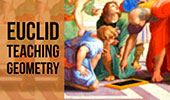# Online Geometry: Triangle Centers, Theorems and Problems Index, Page 5

 Triangle Centers - Table of Content 5Geometric art of triangle centers visual summary Delaunay Triangulation, Circular Random Composition, iPad Apps. Problem 32. Triangle, Cevian, Incircles, Tangents. Problem 31. Right Triangle, Incircle, Collinears. Problem 30. Right Triangle, Incircle, Inradius. Proposed Problem 29. Right Triangle, altitude, incircle and inradius. Ten conclusions. Problem 29: Geometry Help. Facts you should know. Proposed Problem 28 Right Triangle, altitude, incircles and inradius. Proposed Problem 27 Right Triangle, incircles and inradius. Proposed Problem 26 Right Triangle, altitude, incircles and inradius. Proposed Problem 25 Right Triangle, altitude, incircles and inradius. Proposed Problem 24 Right Triangle, altitude, incircles and inradii. Proposed Problem 23 Right Triangle, altitude, incircles and inradii. Proposed Problem 22 Right Triangle, altitudes, incircles and inradii. Proposed problem 21 Acute triangle, orthocenter, diameter, tangents. Proposed problem 20 Right Triangle, incircles and inradii. Proposed problem 19 Right Triangle and Excenter Heron's Formula. Key facts and a purely geometric step-by-step proof. Napoleon's Theorem. A purely geometric proof. It uses the Fermat point to prove Napoleon without transformations.

Go to Page: Previous | 1 | 2 | 3 | 4 | 5 | Next

 Home | Sitemap | Search | Geometry | Email | by Antonio Gutierrez